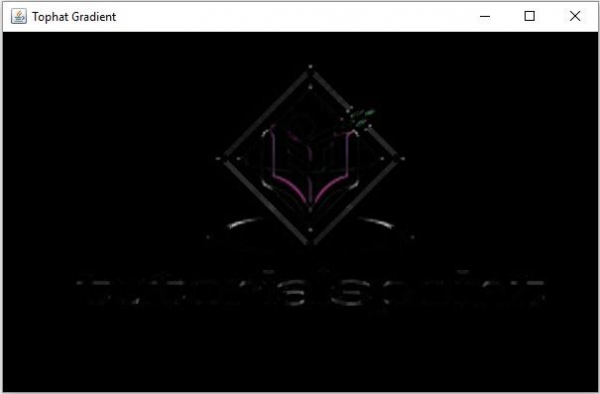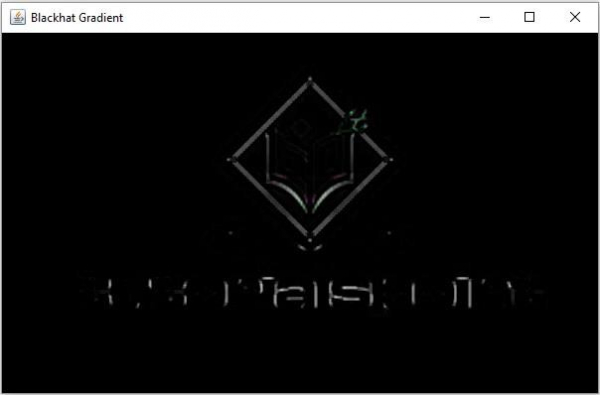# Explain Top Hat and Black hat morphological operations in Java.

Morphological operations are the set of operations that process images according to the given shapes.

• Erosion − Erosion is a morphological operation during which pixels are removed from the image boundaries.

• Dilation − During is a morphological operation during which pixels are added to the image boundaries.

• Where the total number of pixels added/removed depends on the dimensions of the structuring element used.

• Morphological Opening − During this operation erosion is applied on the given input and on the result dilation is applied. This is used to remove small objects from the foreground of an image.

• Morphological Closing − During this operation dilation is applied on the given input and on the result erosion is applied. This is used to remove small objects on an image.

A Morphological Top Hat is a difference between the given image and its opening.

## Example

import org.opencv.core.Core;
import org.opencv.core.CvType;
import org.opencv.core.Mat;
import org.opencv.highgui.HighGui;
import org.opencv.imgcodecs.Imgcodecs;
import org.opencv.imgproc.Imgproc;
public class TopHatExample {
public static void main(String args[]) {
String file ="D:\Images\morph_input1.jpg";
//Creating destination matrix
Mat dst = new Mat(src.rows(), src.cols(), src.type());
//Preparing the kernel matrix object
Mat kernel = Mat.ones(5,5, CvType.CV_32F);
//Applying dilate on the Image
Imgproc.morphologyEx(src, dst, Imgproc.MORPH_TOPHAT, kernel);
//Displaying the image
HighGui.waitKey();
}
}

## Input Image## OutputA Morphological Black Hat is a difference between the closing and the given image.

## Example

import org.opencv.core.Core;
import org.opencv.core.CvType;
import org.opencv.core.Mat;
import org.opencv.highgui.HighGui;
import org.opencv.imgcodecs.Imgcodecs;
import org.opencv.imgproc.Imgproc;
public class TopHatExample {
public static void main(String args[]) {
String file ="D:\Images\morph_input1.jpg";
//Creating destination matrix
Mat dst = new Mat(src.rows(), src.cols(), src.type());
//Preparing the kernel matrix object
Mat kernel = Mat.ones(5,5, CvType.CV_32F);
//Applying dilate on the Image
Imgproc.morphologyEx(src, dst, Imgproc.MORPH_BLACKHAT, kernel);
//Displaying the image
HighGui.waitKey();
}
}

## Input Image## Output